1. # FLWOR Expressions

As we have already seen in the first chapter, FLWOR expressions are functionally equivalent to SQL SELECT statements.

FLWOR expressions enable you to do several things including:

• iterating over sequences
• joining multiple documents
• grouping/aggregating

The fundamental building block of FLWOR expressions is the clause.

1. ## FLWOR Expression Clauses

A FLWOR expression can contain up to eight different clauses:

• for
• let
• where
• order by
• return
• count
• group by
• window

FLWOR is an acronym made up of the first letter of five of these clauses: 'For', 'Let', 'Where', 'Order by' and 'Return'.

A FLWOR expression must always begin with the keyword for or let.

A FLWOR expression must always end with a return clause.

The for and let clauses begin with the keyword for and let respectively. The window clause does not begin with the keyword window, instead it begins with the keyword for (like the for clause).

The next sections will describe the FLWOR clauses and provide examples showing their usage.

1. ### for

The for clause is used to iterate over items in a sequence.

In its most basic form, the for clause begins with the keyword for followed by a variable declaration (e.g. \$x). The variable declaration is followed by the keyword in, followed by an expression which evaluates to a sequence of items.

The variable iterates over the sequence and is bound to each item in turn.

example: for clause iterating over nodes in source xml document```for \$i in /people/person
return \$i/name```
result:
```<name>mary</name>
<name>cindy</name>
<name>john</name>
<name>peter</name>```
• The for clause in this basic example declares and binds the variable \$i to the sequence of person elements which are children of the root element people and then iterates over each item in turn.
• The return clause returns the name child element of each \$i.

example: for clause iterating over sequence of integers

```for \$i in (1 to 5)
return \$i```
result:
```1
2
3
4
5```
• The for clause in this example binds a variable \$i to the sequence of items constructed by the range (1 to 5) i.e. the integers 1, 2, 3, 4 and 5 and then iterates over each item in the sequence.
• The return clause simply returns each of these items.

example: multiple for clauses iterating over different sequences

```for \$x in (1, 2)
for \$y in ('a', 'b')
return concat (\$x, \$y)```
result:
```'1a'
'1b'
'2a'
'2b'```
• The two for clauses in this example bind the variable \$x to the sequence of items (1, 2) and \$y to the sequence of items ('a', 'b').
• The return clause returns the concatenation of \$x and \$y.

example: multiple variable bindings in one for clause

```for \$x in (1, 2), \$y in ('a', 'b')
return concat (\$x, \$y)```
result:
```'1a'
'1b'
'2a'
'2b'```
This example is equivalent to the previous one but more elegant. Instead of declaring the for keyword twice, a comma is used to separate the two variable bindings.
2. ### let

The let clause is used to bind further values to variables which will be used later in the FLWOR expression.

The keyword let is followed by the variable name e.g. \$a, followed by := and the value which is to be bound to the variable.

example: let clause binding variable to node in source xml document```for \$i in /people/person
let \$a := \$i/age
return \$a```
result:
```<age>20</age>
<age>25</age>
<age>40</age>
<age>35</age>```
• The for clause in this example binds the variable \$i to /people/person and then iterates over each item in the sequence.
• The let clause binds the variable \$a to \$i/age (where age is a child element of /people/person).
• The return clause returns \$a i.e. the age child element of each \$i.

example: multiple variable bindings in one let clause```for \$i in /people/person
let \$a := \$i/age, \$n := \$i/name
return concat (\$n, ' is ', \$a)```
result:
```'mary is 20'
'cindy is 25'
'john is 40'
'peter is 35'```
• The for clause in this example binds the variable \$i to /people/person and then iterates over each item in the sequence.
• The let clause declares two variables \$a and \$n. Each variable assignment is separated by a comma.
• The return clause returns the concatenation of \$n, the string 'is ', and \$a.
3. ### where

The where clause is used to filter the input stream.

The keyword where is followed by an expression which returns a boolean value. Only those tuples for which the boolean expression returns true are filtered.

Tuples are explained after the next example.

example: where clause filtering nodes from source xml document```for \$i in /people/person
let \$a := \$i/age, \$n := \$i/name
where \$a < 30
return \$n```
result:
```<name>mary</name>
<name>cindy</name>```
• The for clause in this example binds the variable \$i to /people/person and then iterates over each item in the sequence.
• The let clause declares two variables \$a and \$n. Each variable assignment is separated by a comma.
• The where clause filters the /people/person nodes to those person elements with a child element named age which has a value less than 30.
• The return clause returns the name child elements of the filtered /people/person tuples.
1. #### tuples

Tuples are best understood by example: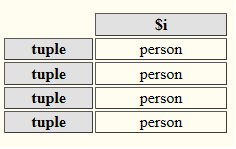`for \$i in /people/person`
• The for clause creates a tuple for each item in the binding sequence.
• The variable \$i is bound to each item in turn.
• As there are four person elements which are children of the root element people in the source file, the tuple stream contains four tuples at this point in the FLWOR expression.
• Each tuple can be thought of as a row in a table known as the tuple space.
• Each variable in the FLWOR expression can be thought of as a column in the tuple space table.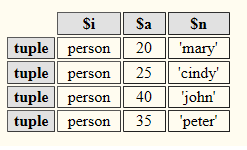`let \$a := \$i/age, \$n := \$i/name`
• The let clause binds the variables \$a and \$n to \$i/age and \$i/name respectively.
• The tuple space table contains an additional two columns for \$a and \$n respectively.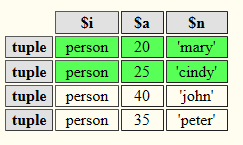```let \$a := \$i/age, \$n := \$i/name
where \$a < 30```
The where clause filters the tuple stream (i.e. the existing tuples at this point in the FLWOR expression) to only those tuples for which the value of \$n is less than 30.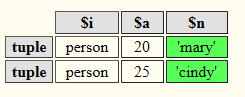```let \$a := \$i/age, \$n := \$i/name
where \$a < 30
return \$n```
The return clause returns the value of the variable \$n for each of the remaining tuples in the tuple stream. i.e. the names 'mary' and 'cindy'.
4. ### order by

The order by clause is used for value based ordering.

The keywords order by are followed by the item (key) used to order the tuple stream.

The qualifiers ascending or descending can be used to indicate the sort order.

example: order by clause sorting filtered sequence in ascending order```for \$i in /people/person
let \$a := \$i/age, \$n := \$i/name
where \$a < 30
order by \$n ascending
return \$n```
result:
```<name>cindy</name>
<name>mary</name>```
• The for clause in this example binds the variable \$i to /people/person and then iterates over each item in the sequence.
• The let clause declares two variables \$a and \$n. Each variable assignment is separated by a comma.
• The where clause filters the input stream to those /people/person elements with a child element named age which has a value less that 30.
• The order by clause orders the filtered input stream using the value of the name child element as the sort key (If no qualifier is specified, the default sort order is ascending).
• The return clause returns the name elements in ascending order (i.e. 'a' is sorted before 'z').
5. ### count

The count clause is used to declare a new variable which is bound to the ordinal position of the respective tuple in the tuple stream providing a mechanism to enumerate the tuples.

The keyword count is followed by a variable declaration e.g. \$x, which is bound to each tuple in turn.

example: count clause binding variable to each item of input sequence```for \$i in /people/person
let \$n := \$i/name
count \$x
return concat (\$x, '. ', \$n)```
result:
```'1. mary'
'2. cindy'
'3. john'
'4. peter'```
• The for clause in this example binds the variable \$i to /people/person and then iterates over each item in the sequence.
• The let clause declares and binds the variable \$n to \$i/name.
• The count clause binds the variable \$x to the tuple stream, which in this case consists of each person element which is a child of people. This variable is incremented for each person (tuple) in the stream.
• The return clause concatenates the value of the variable \$x with a string '. ' and the value of the variable \$n for each tuple.

example: count clause binding variable to each item of filtered input sequence```for \$i in /people/person
let \$a := \$i/age, \$n := \$i/name
where \$a < 30
count \$x
return concat (\$x, '. ', \$n)```
result:
```'1. mary'
'2. cindy'```
• The for clause in this example binds the variable \$i to /people/person and then iterates over each item in the sequence.
• The let clause declares and binds the variables \$a to \$i/age and \$n to \$i/name.
• The where clause filters the input stream to those /people/person elements with an age child element which has a value less than 30.
• The count clause binds the variable \$x to the /people/person elements filtered by the where clause. The variable is incremented for each person in the stream with an age less than 30.
• The return clause concatenates the value of the variable \$x with a string '. ' and the value of the variable \$n for each tuple.
6. ### group by

The group by clause is used to group tuples from the input stream which share the same grouping key value.

The keywords group by are followed by an expression representing the grouping key.

example: group by clause grouping input sequence by department```<departments>
{
for \$i in /people/person
let \$n := <employee>{data(\$i/name)}</employee>, \$d := \$i/@dept
group by \$d
return <department name="{\$d}">
{
\$n
}
</department>
}
</departments>```
result:
```<departments>
<department name="accounting">
<employee>peter</employee>
</department>
<department name="marketing">
<employee>cindy</employee>
</department>
<department name="sales">
<employee>mary</employee>
<employee>john</employee>
</department>
</departments>```
• This example begins with the start tag of a direct element constructor for departments i.e. <departments> and ends with the end tag i.e. </departments>.
• The direct element constructor for departments includes an enclosed expression contained within an opening { and closing } for the element contents.
• The for clause of the FLWOR expression binds the variable \$i to the sequence of items selected by the /people/person XPath expression and then iterates over each item in the sequence.
• The let clause declares two variables. The first variable \$n is bound to a direct element constructor for employee. The second variable \$d is bound to the dept attribute of the person element.
• The group by clause groups the input stream of /people/person elements using the value of the dept attribute as the grouping key.
• The return clause uses a direct element constructor to output a department element for each group. Within each department, the respective employee elements are generated from the value of the name element belonging to the respective person in the group being processed.
7. ### window

The window clause is similar to the for clause in that it too iterates over a binding sequence.

The window clause derives zero or more windows from the binding sequence.

Each window is a sequence of consecutive items from the binding sequence.

The number of windows and the items occuring in each window are determined by the type of window as well as specific constraints.

Constraints are specified in the start and end sections of the window clause.

Constraints use window variables and conditional logic to determine where each window generated from the binding sequence begins and ends.

1. #### first line

Much like a for clause, the first line of a window clause begins with the keyword for.

for is followed by a declaration of which type of window(s) will be generated from the binding sequence. There are two types of window: tumbling windows or sliding windows.

The type of window is followed by a user defined variable e.g. \$w representing the window itself.

The user defined variable representing the window is followed by the keyword in and the binding sequence from which the window(s) will be derived.

`for tumbling window \$w in ('A', 'B', 'BB', 'BBB', 'C', 'CC', 'CCC', 'CCCC')`
2. #### start constraint

The start constraint is the second line of the window clause.

As its name implies, the start constraint defines where a window can start.

The start constraint begins with the keyword start followed by window variables e.g \$s. The window variables are then followed by the keyword when.

The keyword when is followed by a boolean expression. If the expression returns true the particular item in the binding sequence currently being processed is a candidate for the start of a window (subject to the type of window: tumbling or sliding specified in the first line).

```for tumbling window \$w in ('A', 'B', 'BB', 'BBB', 'C', 'CC', 'CCC', 'CCCC')
start \$s when string-length(\$s) = 1```

In the example above a window can only start with the item being processed if that item is one character long.

3. #### end constraint

The end constraint is optional, if it is specified it appears after the start constraint.

As its name implies, the end constraint defines where a window can end.

The end constraint begins with the keyword end followed by window variables e.g \$e. The window variables are then followed by the keyword when.

The keyword when is followed by a boolean expression. If the expression returns true the particular item in the binding sequence currently being processed is a candidate for the end of a window (subject to the type of window: tumbling or sliding specified in the first line).

```for tumbling window \$w in ('A', 'B', 'BB', 'BBB', 'C', 'CC', 'CCC', 'CCCC')
start \$s when string-length(\$s) = 1
end \$e when string-length(\$e) = 2```

In the example above a window can only end with the item being processed if that item is two characters long.

4. #### return

The return clause of a window clause returns the windows generated from the binding sequence.

```for tumbling window \$w in ('A', 'B', 'BB', 'BBB', 'C', 'CC', 'CCC', 'CCCC')
start \$s when string-length(\$s) = 1
end \$e when string-length(\$e) = 2
return <window>
{
for \$i in \$w
return <item>{\$i}</item>
}
</window>```

In the example above the return statement creates a literal window element for each generated window.

Within the literal window element's opening and closing tags is a for clause which iterates through each item in the current window being processed.

The result of the window clause in this example is two windows.

The first window contains three items 'A' , 'B', and 'BB'.

The second window contains two items 'C' and 'CC'.

```<window>
<item>A</item>
<item>B</item>
<item>BB</item>
</window>
<window>
<item>C</item>
<item>CC</item>
</window>```
5. #### window types

There are two types of window:

• tumbling
• sliding

Tumbling windows are windows which never overlap one another. This means that the start item of a new window can only be an item in the binding sequence which occurs after the last item in a previous window (if a previous window exists).

The image below shows two tumbling windows. The first window contains the first three items in the sequence i.e A, B and C . The second window contains two items D and E.

In this particular example the second window starts with the fourth item in the binding sequence, which is the item occuring immediately after the last item in the first window.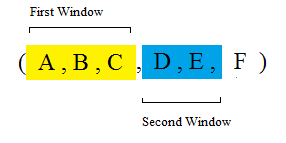Sliding windows are windows which can overlap one another. This means that the start item of a new window can be an item in the binding sequence which is the last item or occurs before the last item of the previous window (if a previous window exists).

The image below shows two sliding windows. The first window contains the first three items in the binding sequence i.e A, B and C . The second window contains three items C, D and E.

In this particular example the second window starts with the third item in the binding sequence i.e. C, which is also the last item in the first window. The windows therefore overlap.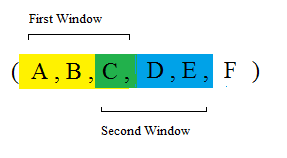The two examples below demonstrate the difference between tumbling and sliding windows. The window clause in each example is indentical except for the type of window being generated:

example: tumbling window

```  for tumbling window \$w in ('A', 'B', 'BB', 'BBB', 'C', 'CC', 'CCC', 'CCCC')
start \$s when string-length(\$s) = 1
end \$e when string-length(\$e) = 2
return <window>
{
for \$i in \$w
return <item>{\$i}</item>
}
</window>```
result:
```<window>
<item>A</item>
<item>B</item>
<item>BB</item>
</window>
<window>
<item>C</item>
<item>CC</item>
</window>```
• In this tumbling window example two windows are generated.
• The first window has three items 'A', 'B' and 'BB'.
• The second window has two items 'C' and 'CC'.

example: sliding window

```  for sliding window \$w in ('A', 'B', 'BB', 'BBB', 'C', 'CC', 'CCC', 'CCCC')
start \$s when string-length(\$s) = 1
end \$e when string-length(\$e) = 2
return <window>
{
for \$i in \$w
return <item>{\$i}</item>
}
</window>```
result:
```<window>
<item>A</item>
<item>B</item>
<item>BB</item>
</window>
<window>
<item>B</item>
<item>BB</item>
</window>
<window>
<item>C</item>
<item>CC</item>
</window>```
• In this sliding window example three windows are generated.
• The first window has three items 'A', 'B' and 'BB'.
• The second window has two items 'B' and 'BB'.
• The third window has two items 'C' and 'CC'.
6. #### window variables

There are a total of nine different window variables.

In conjunction with the type of window, constraints which make use of window variables determine how many windows will be generated from the binding sequence and which items from the binding sequence will be in each window.

A window can be represented by at least one and at most nine of these variables.

Only one variable is actually mandatory, that being the variable which represents the window itself (the variable declared after the "window type" in the first line of the window clause).

Each variable fulfils a specific role. We have already encountered three of these variables representing the window itself, the start-item and end-item roles.

The nine roles associated with the variables in a window clause are:

• window-variable: bound to the sequence of items from the binding sequence which comprise the window.
• start-item: bound to the first item in the window.
• start-item-position: bound to the position of the first window item in the binding sequence.
• start-previous-item: bound to the item in the binding sequence which precedes the first item in the window.
• start-next-item: bound to the item in the binding sequence which follows the first item in the window.
• end-item: bound to the last item in the window.
• end-item-position: bound to the position of the last window item in the binding sequence.
• end-previous-item: bound to the item in the binding sequence which precedes the last item in the window.
• end-next-item: bound to the item in the binding sequence which follows the last item in the window.

The following examples will demonstrate use of several of these roles as well as use of the keyword only.

example: tumbling window demonstrating start-item role

```<windows>
{
for tumbling window \$w in ('A', 'B', 'BB', 'BBB', 'C', 'CC', 'CCC', 'CCCC')
start \$s when string-length(\$s) = 1
return <window>
{
for \$i in \$w
return <item>{\$i}</item>
}
</window>
}
</windows>```
result:
```<windows>
<window>
<item>A</item>
</window>
<window>
<item>B</item>
<item>BB</item>
<item>BBB</item>
</window>
<window>
<item>C</item>
<item>CC</item>
<item>CCC</item>
<item>CCCC</item>
</window>
</windows>```
• The for clause in this example binds the variable \$w to the sequence of items ('A', 'B', 'BB', 'BBB', 'C', 'CC', 'CCC', 'CCCC') and then iterates over each item in the sequence.
• The start condition uses the start-item role to specify that a window can only be generated if the item in question is of length = 1. The start-item role is indicated by the keyword start.

example: tumbling window demonstrating start-item and end-item roles

```<windows>
{
for tumbling window \$w in ('A', 'B', 'BB', 'BBB', 'C', 'CC', 'CCC', 'CCCC')
start \$s when string-length(\$s) = 1
end \$e when string-length(\$e) = 2
return <window>
{
for \$i in \$w
return <item>{\$i}</item>
}
</window>
}
</windows>```
result:
```<windows>
<window>
<item>A</item>
<item>B</item>
<item>BB</item>
</window>
<window>
<item>C</item>
<item>CC</item>
</window>
</windows>```
• This example demonstrates use of the start-item and end-item roles. The start condition specifies that the start of a window can only occur if an item's value is of length = 1
• The end condition specifies that the end of the window should occur if an item's value is of length = 2.

example: tumbling window demonstrating start-item-position and end-item-position roles

```<windows>
{
for tumbling window \$w in ('A', 'B', 'C', 'D', 'E', 'F', 'G')
start at \$s when true()
end at \$e when \$e - \$s eq 2
return <window>
{
for \$i in \$w
return <item>{\$i}</item>
}
</window>
}
</windows>```
result:
```<windows>
<window>
<item>A</item>
<item>B</item>
<item>C</item>
</window>
<window>
<item>D</item>
<item>E</item>
<item>F</item>
</window>
<window>
<item>G</item>
</window>
</windows>```
• This example demonstrates tumbling windows. The windows are defined by start and end conditions which are specified using variables representing the start-item-position and end-item-position roles respectively.
• The for clause in this example binds the variable \$w to the sequence of items ('A', 'B', 'C', 'D', 'E', 'F', 'G') and then iterates over each item in the sequence.
• The start condition begins with the keywords start at (indicating the start-item-position role) followed by a user defined variable \$s.
• The user defined variable \$s is followed by the keyword when, followed by a boolean expression for which the start-item-position is valid. In this case the boolean expression is simply a call to the true() function (which always returns true). This means that any item in the binding sequence could potentially be the start of a new window (subject to other constraints on the window, i.e type of window, end condition parameters etc.).
• The end condition begins with the keywords end at (indicating the end-item-position role) followed by a user defined variable \$e.
• The user variable \$e is followed by the keyword when, followed by a boolean expression for which the end-item-position is valid. The boolean expression in this case returns true if the end item's position minus the start item's position is equal to 2 i.e. \$e - \$s = 2
• This XQuery expression generates three windows.
• The first window starts at the item in first position in the sequence i.e. 'A' and ends with item 'C' because 'C' is at position 3 i.e. (3 - 1 = 2). The first window contains items 'A', 'B' and 'C'.
• The second window starts at the position after the last item in the first window i.e item 'D' at position 4 in the sequence, and ends with item 'F' because 'F' is at position 6 i.e. (6 - 4 = 2). The second window contains items 'D', 'E' and 'F'.
• A third window is also created. The third window starts at the position after the last item in the second window i.e. item 'G' at position 7 in the sequence.. Because there are no other items in the sequence after this item, the window will also end here even though the end condition has not been met i.e. 7 - 7 is not equal to 2.

example: tumbling window demonstrating start-item-position and end-item-position roles, and 'only' keyword

```<windows>
{
for tumbling window \$w in ('A', 'B', 'C', 'D', 'E', 'F', 'G')
start at \$s when true()
only end at \$e when \$e - \$s eq 2
return <window>
{
for \$i in \$w
return <item>{\$i}</item>
}
</window>
}
</windows>```
result:
```<windows>
<window>
<item>A</item>
<item>B</item>
<item>C</item>
</window>
<window>
<item>D</item>
<item>E</item>
<item>F</item>
</window>
</windows>```
• This example demonstrates use of the only keyword which is used to specify that a window should only be created if the end condition is also met.
• This example is identical to the previous one except for the keyword only which appears at the beginning of the end condition.
• As opposed to the previous example in which a third window (for item 'G' )was generated although the end condition was not met i.e. end-item-position minus start-item-position was not equal to 2 i.e. (7 - 7 = 0).
• This example specifies that a window should only be created if the end condition is also met and as such only generates two windows, the first with items 'A', 'B' and 'C' and the second with items 'D', 'E', and 'F'.

example: sliding window demonstrating start-item-position and end-item-position roles and 'only' keyword

```<windows>
{
for sliding window \$w in ('A', 'B', 'C', 'D', 'E', 'F', 'G')
start at \$s when true()
end at \$e when \$e - \$s eq 2
return  <window>
{
for \$i in \$w
return <item>{\$i}</item>
}
</window>
}
</windows>```
result:
```<windows>
<window>
<item>A</item>
<item>B</item>
<item>C</item>
</window>
<window>
<item>B</item>
<item>C</item>
<item>D</item>
</window>
<window>
<item>C</item>
<item>D</item>
<item>E</item>
</window>
<window>
<item>D</item>
<item>E</item>
<item>F</item>
</window>
<window>
<item>E</item>
<item>F</item>
<item>G</item>
</window>
<window>
<item>F</item>
<item>G</item>
</window>
<window>
<item>G</item>
</window>
</windows>```
• This example is the same as the second tumbling window example except it is a sliding window.
• Because the start condition always evaluates to true, each item in the input sequence starts a new window resulting in seven windows.

example: tumbling window demonstrating start-item, end-item and end-next-item roles

```<windows>
{
for tumbling window \$w in ( 'A', 'B', 'B', 'A', 'A', 'C', 'D', 'D', 'D', 'E', 'A',  'A')
start \$s when true()
end \$e next \$e-next when \$e-next != \$e
return <window>
{
for \$i in \$w
return <item>{\$i}</item>
}
</window>
}
</windows>```
result:
```<windows>
<window>
<item>A</item>
</window>
<window>
<item>B</item>
<item>B</item>
</window>
<window>
<item>A</item>
<item>A</item>
</window>
<window>
<item>C</item>
</window>
<window>
<item>D</item>
<item>D</item>
<item>D</item>
</window>
<window>
<item>E</item>
</window>
<window>
<item>A</item>
<item>A</item>
</window>
</windows>```
• This example demonstrates use of the start-item, end-item and the end-next-item roles.
• The start condition specifies that the start of a window can occur with any item in the sequence.
• The end condition specifies that the end of a window occurs only if the next item in the sequence is different from the current item i.e. \$e-next != \$e.

example: tumbling window demonstrating start-item, end-item, start-next-item, end-next-item and end-previous-item roles

```<windows>
{
for tumbling window \$w in ( 'A', 'B', 'B', 'A', 'A', 'C', 'D', 'D', 'D', 'E', 'A',  'A')
start \$s next \$s-next when  \$s = \$s-next
end \$e previous \$e-previous next \$e-next when \$e-previous = \$e and \$e-next != \$e
return <window>
{
for \$i in \$w
return <item>{\$i}</item>
}
</window>
}
</windows>```
result:
```<windows>
<window>
<item>B</item>
<item>B</item>
</window>
<window>
<item>A</item>
<item>A</item>
</window>
<window>
<item>D</item>
<item>D</item>
<item>D</item>
</window>
<window>
<item>A</item>
<item>A</item>
</window>
</windows>```
• This example demonstrates use of the start-item, end-item, start-next-item, end-next-item and end-previous-item roles.
• The effect that the specified start and end conditions have is to generate a window for each sub-sequence of two or more consecutive items with the same value from the binding sequence.